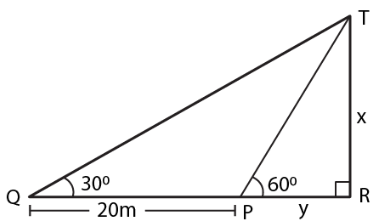Guru

# A person standing on the bank of a river observes that the angle subtended by a tree on the opposite bank is 600; when he retires 20 m from the bank, he finds the angle to be 300. Find the height of the tree and the breadth of the river.

• 0

sir this is the question from the book -ML aggarwal( avichal publication) class 10th , chapter20 , heights and distances
this question once has been asked in exam . and have chances to come in  future .
we know A person standing on the bank of a river observes that the angle subtended by a tree on the opposite bank is 600;
when he retires 20 m from the bank,
he finds the angle to be 300.
wehave to Find the height of the tree and the breadth of the river.
question no 17 , heights and distances , ICSE board

Share

1. Consider TR as the tree and PR as the width of the river.Take TR = X and PR = y

In right triangle TPR

tan θ = TR/PR

Substituting the values

tan 600 = x/y

So we get

√3 = x/y

x = y √3…… (1)

In right triangle TQR

tan 300 = TR/QR

tan 300 = x/(y + 20)

We get

1/√3 = x/(y + 20)

x = (y + 20)/ √3 ….. (2)

Using both the equations

y√3 = (y + 20)/ √3

So we get

3y = y + 20

3y – y = 20

2y = 20

y = 10

Now substituting the value of y in equation (1)

x = 10 × √3 = 10 (1.732) = 17.32

Hence, the height of the tree is 17.32 m and the width of the river is 10 m.

• 0